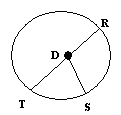Name: ___________________Date:___________________

 Email us to get an instant 20% discount on highly effective K-12 Math & English kwizNET Programs!

### Grade 4 - Mathematics4.5 Circle Facts - 2

 Circles are simple closed curves which divide the plane into an interior and exterior. Circle is a closed figure and not a polygon. A circle can be drawn with the help of a compass. All the points on a circle are at the same distance from the center of the circle. A line from the center to any point on the circle is called radius. Diameter is twice the radius. The circumference of a circle means the length of the circle. Directions: Answer the following. Draw a circle with compass and write all its properties.Name: ___________________Date:___________________

### Grade 4 - Mathematics4.5 Circle Facts - 2

 Q 1: All the points on a circle are at the same distance from thecenter of the circlepoints outside the circlepoints on the circle Q 2: All the radii of a circle have thedifferent lengthssame lengths Q 3: In the circle shown what the segments of equal lengthDT, DR and DSDR, DR and DSDT, TR and DS Q 4: The diameter of a circle ishalf the radiustwice the radiussame as radius Q 5: Circle is a closed figure and is not a ________squarequadrilateralpolygon Q 6: The radius of a circle is alwayssame as diameterhalf the diametertwice the diameter Question 7: This question is available to subscribers only! Question 8: This question is available to subscribers only!